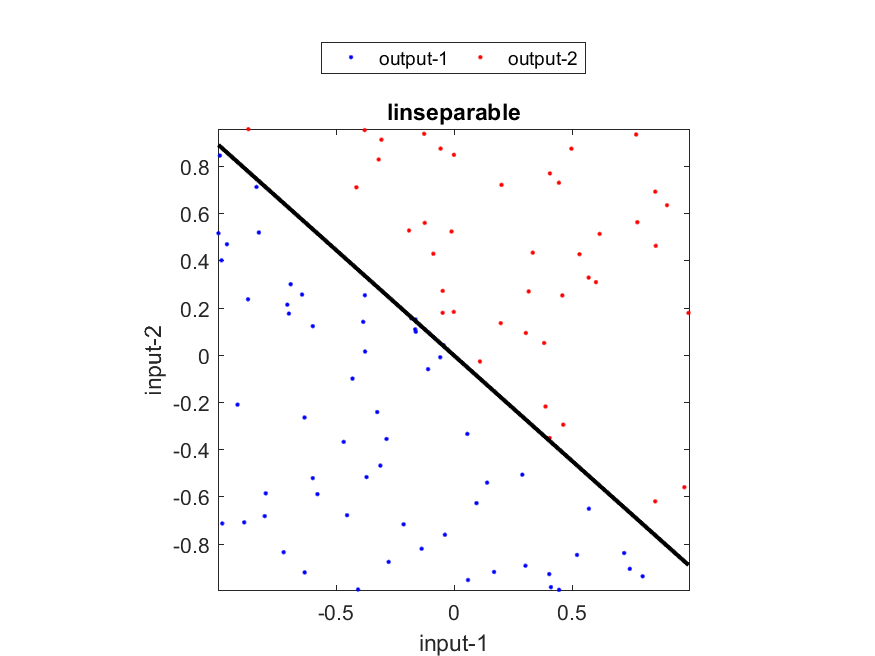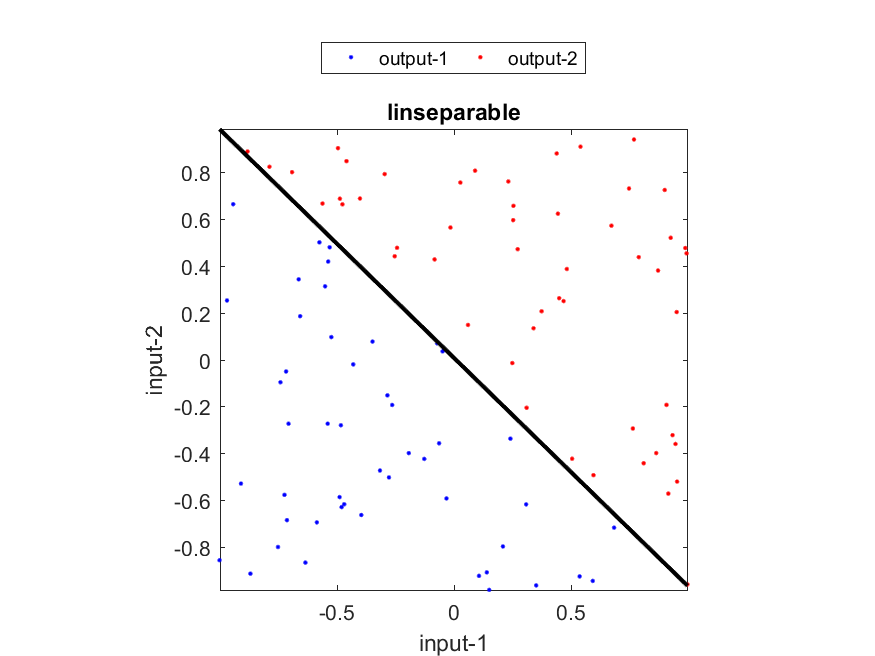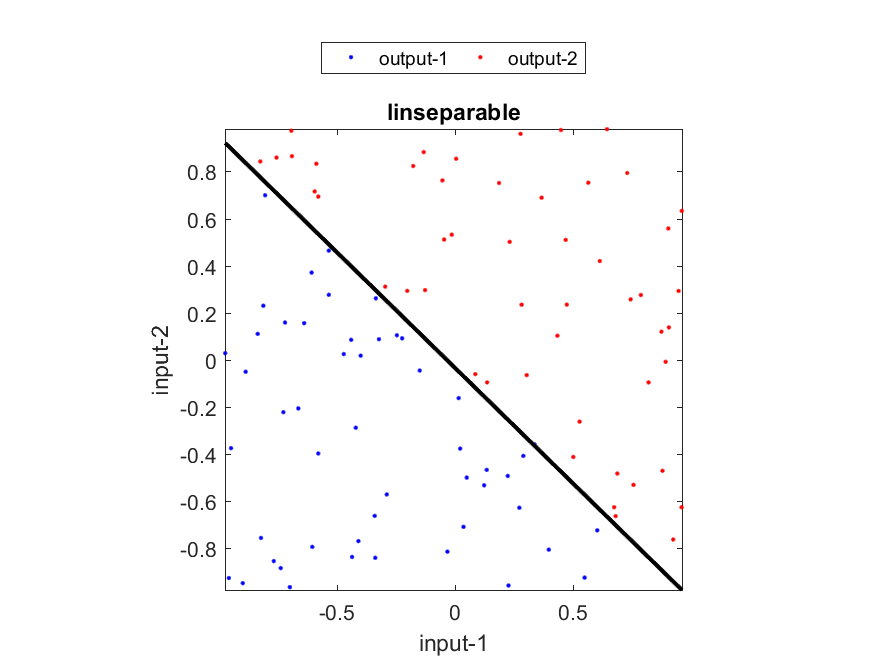5-4 Linear Classifiers (嚙踝蕭嚙踝蕭嚙踝蕭嚙踝蕭嚙踝蕭嚙踝蕭嚙踝蕭嚙?

The linear classifier uses a linear function to map feature vectors into a hyperplane. If the hyperplane is higher than a threshold, then the corresponding feature vectors belong to one class. Otherwise, they belong to the other class. By nature, the linear classifier is suitable for 2-class problems.

Assuming the feature space has a dimension of 2, the linear classifier implements a plane in the 3D space. If a feature vector is denoted as x=[x1, x2], then the function implemented by the linear classifier can be defined as

f(x, w) = sgn(w1x1 + w2x2 + w3)
If the output y is -1, then x is class 1. Otherwise it is class 2. (Note that for simplicity, we use -1 and 1 to denote two different classes.) The decision boundary of the linear classifier is then located at where the plane is equal to zero:
w1x1 + w2x2 + w3 = 0.

Given a set of training data, the most intuitive way to adapt the paramters (or weights) of the linear classifier is based on Widro's learning. The learning scheme is sequential, which means that the weigths are updated once a given input-output pair (feature vector and its desired class) becomes available sequentially. If we use [x; y] as the feature vector and its class (-1 or 1), then the update rule is

Dw = hw(y-f(x,w))
where h is a small positive number called the learning rate. The weight is updated whenever a training pair is available. It can be proved that the learning rule is guaranteed to converge to a set of weights that will perfectly classify all the data if such a solution exists.

We can use lincTrain.m to train a linear classifier by such sequential learning, as shown below.

Example 1: lincTrain01.mDS=prData('linSeparable'); trainPrm=lincOptSet('method', 'sequentialLearning', 'maxIter', 2000, 'animation', 'yes'); [coef, recogRate]=lincTrain(DS, trainPrm); fprintf('Recog. rate = %.2f%%\n', 100*recogRate);Iteration=200/2000 Iteration=400/2000 Iteration=600/2000 Iteration=800/2000 Iteration=1000/2000 Iteration=1200/2000 Iteration=1400/2000 Iteration=1600/2000 Iteration=1800/2000 Iteration=2000/2000 Recog. rate = 98.00%It is also possible to have batch learning for linear classifiers. An example is shown next.

Example 2: lincTrain02.mDS=prData('linSeparable'); trainPrm=lincOptSet('method', 'batchLearning', 'animation', 'yes', 'printInterval', 30); [coef, recogRate]=lincTrain(DS, trainPrm); fprintf('Recog. rate = %.2f%%\n', 100*recogRate); Iteration=30/1000, recog. rate=48% Iteration=60/1000, recog. rate=51% Iteration=90/1000, recog. rate=56% Iteration=120/1000, recog. rate=73% Iteration=150/1000, recog. rate=89% Recog. rate = 100.00%It is also possible to apply the least-squares method for linear classifiers. Since the LS method only minimizes the regression error, usually the corresponding classification accuracy is not as good as the above mentioned sequential or batch learning. However, the LS method is very efficient, so it is possible to apply the LS method once to have the initial parameters promptly, and then apply batch learning to further improve the recognition rate. The following example demonstrates the use of the LS method to minimize the regression error.

Example 3: lincTrain03.mDS=prData('linSeparable'); trainPrm=lincOptSet('method', 'minRegressionError', 'animation', 'yes'); [coef, recogRate]=lincTrain(DS, trainPrm); fprintf('Recog. rate = %.2f%%\n', 100*recogRate);Recog. rate = 99.00%Data Clustering and Pattern Recognition (資料分群與樣式辨認)